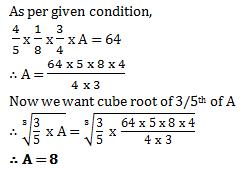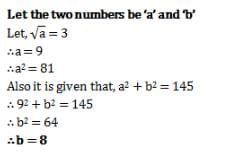# Test: Squares And Square Roots- 2

## 20 Questions MCQ Test NCERT Mathematics for CAT Preparation | Test: Squares And Square Roots- 2

Description
Attempt Test: Squares And Square Roots- 2 | 20 questions in 20 minutes | Mock test for Quant preparation | Free important questions MCQ to study NCERT Mathematics for CAT Preparation for Quant Exam | Download free PDF with solutions
QUESTION: 1

### Four-fifth of one-eighth of 3/4th of A is 64. What is the cube root of 3/5th of A?

Solution:QUESTION: 2

### Find the least number that must be subtracted from 5607 so as to get a perfect square.

Solution:

Square of 75 is 5625 square of 74 is 5476 so use must get 5476

5607 - x is 5476

x is 131

Square root of 5476 is 74

QUESTION: 3

### What will be the number of zeros in the square of 60?

Solution:

The square of 60 is 3600. Therefore, the number of zeroes is 2.

QUESTION: 4

How many natural numbers lie between 92 and 102?

Solution:

Between 92 and 102
Here, n = 9 and n + 1 = 10
∴ Natural number between 92 and 102 are (2 × n) or 2 x 9, i.e. 18.

QUESTION: 5

Find the square of 39.

Solution:

(40-1)= 1600+1-80 = 1521

QUESTION: 6

Without adding, find the sum. 1 + 3 + 5 + 7 + 9 + 11 + 13

Solution:

Total consecutive odd numbers (n) = 7

We know that, Sum = n2

= 72

= 49

Hence, the correct answer is 49

QUESTION: 7

Find the square root of 729.

Solution:

The square root of 729 is 27.

QUESTION: 8

Sum of squares of two numbers is 145. If square root of one number is 3, find the other number.

Solution:QUESTION: 9

If a number has 1 or 9 in the unit’s place, then it’s square ends in ________.

Solution:

If a number has 1 or 9 in the unit’s place, then it’s square ends in 1

QUESTION: 10

None of the square numbers end with _________ at unit’s place.

Solution:

According to the Property 1, A number having 2, 3, 7 or 8 at it's unit's place is never a perfect square. In other words, no square number ends in 2, 3, 7 or 8.

QUESTION: 11

________ is the inverse operation of square.

Solution:

The inverse of squaring a number is square root.

QUESTION: 12

Find the perfect square numbers between 50 and 60.

Solution:

Since we have 72=49 and 82=64.There is no perfect square between 50 and 60.

QUESTION: 13

What is the least perfect square which is divisible by 2, 4 and 6?

Solution:

LCM of 2,4,6= 2×1×2×3=12
Least perfect square which is a multiple of 12 is 36.

QUESTION: 14

2025 plants are to be planted in a garden in such a way that each row contains as many plants as the number of rows. Find the number of plants in each row.

Solution:

Let the number of rows be x
Thus, the each row contains x plants.
The total number of plants in the garden = number of rows × number of plants in each rows
Thus,
2025=x×x
=>x=2025
=>x=  3×3×3×3×5×5
​       =3×3×5
=45
Thus, there are 45 rows and each row contains 45 plants.

QUESTION: 15

Find the greatest 4-digit number which is a perfect square.

Solution:

By division method we find the root of 9999 and we get the remainder 198.So subtracting 198 from 9999 we get 9801 as the greatest 4-digit perfect square.

QUESTION: 16

What will be the number of zeros in the square of 400?

Solution:

Square of 400 = 160000

Hence, the number of zeroes is 4

QUESTION: 17

What is smallest number with which 5400 may be multiplied so that the product is perfect cube?

Solution:

∛(5400 x 5) = 30

QUESTION: 18

The largest perfect square between 4 and 50 is

Solution:

The square of 7 is the largest number between 4 to 50.

QUESTION: 19

Without adding,find the sum of 1+3+5+7+9+11+13+15+17+19

Solution:

Total consecutive odd numbers = 10

Thus n = 10

Therefore sum = n × n

= 10× 10 = 100

QUESTION: 20

Find the square root of 529.

Solution:

Square root of 529 is 23Use Code STAYHOME200 and get INR 200 additional OFF Use Coupon Code